# Unity3D 关于模型变形技术代码实现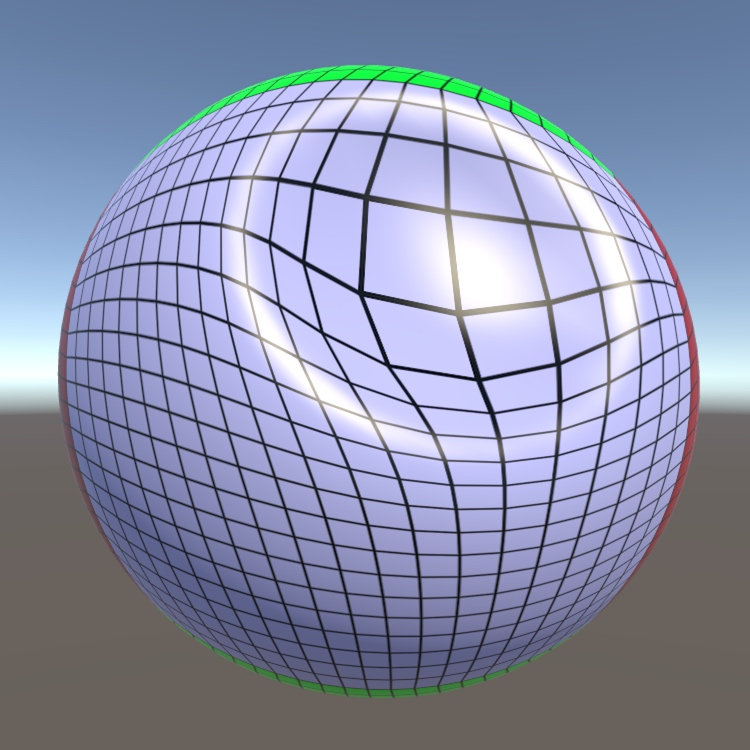# 第一部分准备工作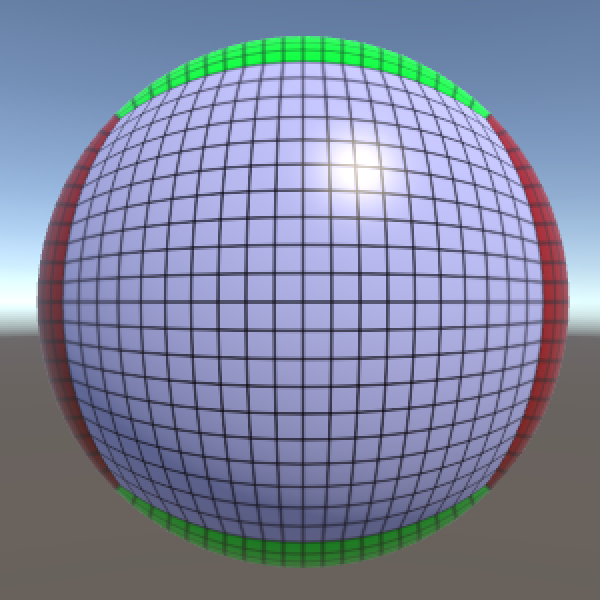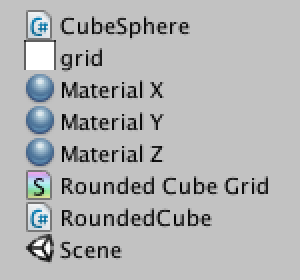using UnityEngine;

[RequireComponent(typeof(MeshFilter))]
public class MeshDeformer : MonoBehaviour {
}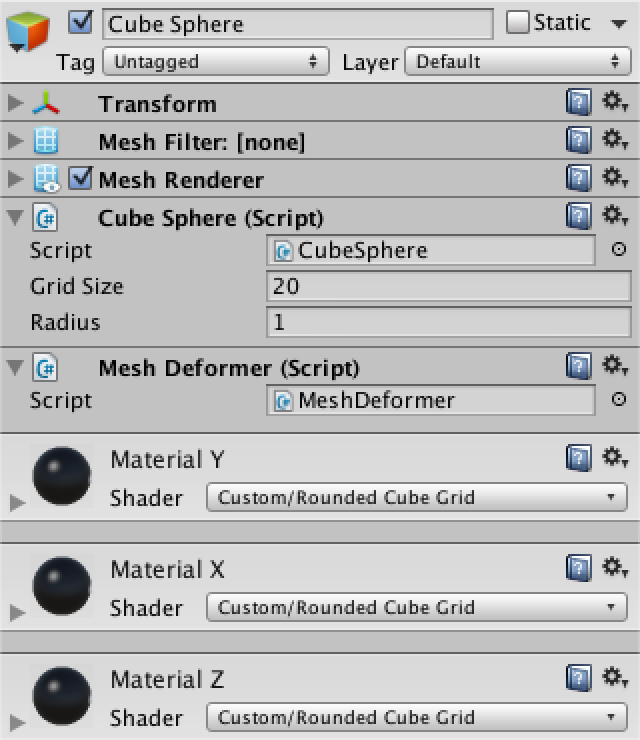Mesh deformingMesh;
Vector3[] originalVertices, displacedVertices;

	void Start () {
deformingMesh = GetComponent<MeshFilter>().mesh;
originalVertices = deformingMesh.vertices;
displacedVertices = new Vector3[originalVertices.Length];
for (int i = 0; i < originalVertices.Length; i++) {
displacedVertices[i] = originalVertices[i];
}
}

	Vector3[] vertexVelocities;

void Start () {
…
vertexVelocities = new Vector3[originalVertices.Length];
}

# 第二部分网格变形输入

using UnityEngine;

public class MeshDeformerInput : MonoBehaviour {

public float force = 10f;
}

在这里要注意，将这个组件附加到相机是最有意义的， 我们不应该将其附加到变形网格物体，因为场景中可能有多个。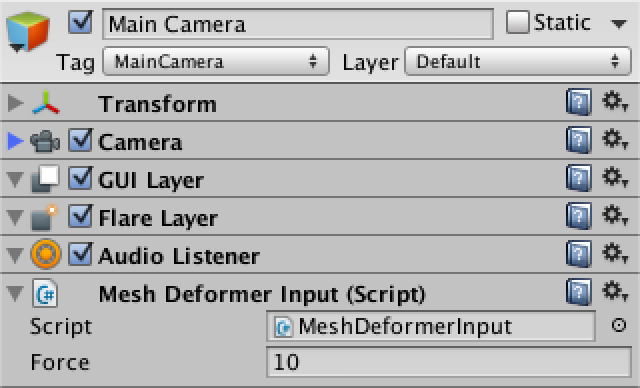具体操作时，当按住默认鼠标按钮时，我们将处理用户的输入， 所以每当有点击或拖动时，只要用户按住。

	void Update () {
if (Input.GetMouseButton(0)) {
HandleInput();
}
}

	void HandleInput () {
Ray inputRay = Camera.main.ScreenPointToRay(Input.mousePosition);
}

		Ray inputRay = Camera.main.ScreenPointToRay(Input.mousePosition);
RaycastHit hit;

if (Physics.Raycast(inputRay, out hit)) {
MeshDeformer deformer = hit.collider.GetComponent<MeshDeformer>();
}

			MeshDeformer deformer = hit.collider.GetComponent<MeshDeformer>();
if (deformer) {
Vector3 point = hit.point;
}

	public void AddDeformingForce (Vector3 point, float force) {
Debug.DrawLine(Camera.main.transform.position, point);
}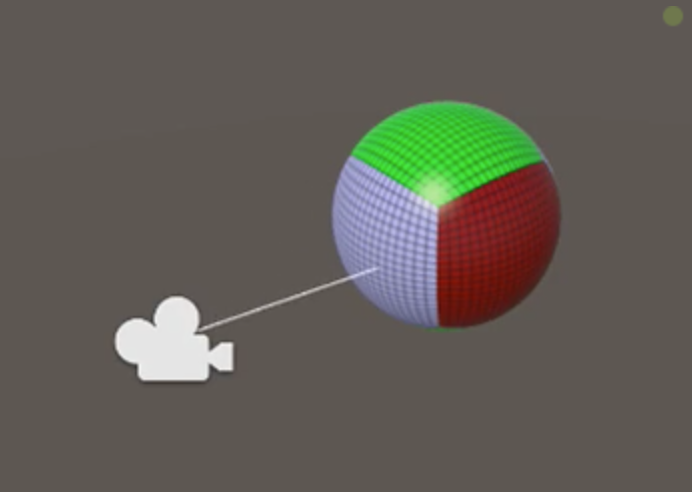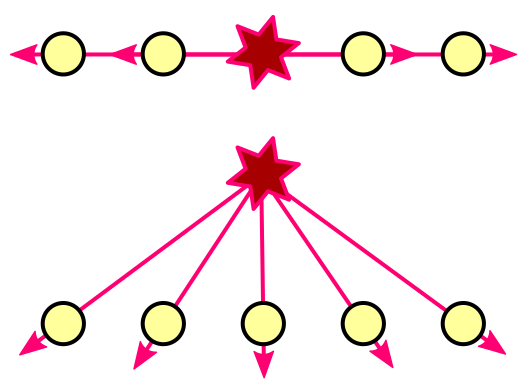public float forceOffset = 0.1f;

void HandleInput () {
Ray inputRay = Camera.main.ScreenPointToRay(Input.mousePosition);
RaycastHit hit;

if (Physics.Raycast(inputRay, out hit)) {
MeshDeformer deformer = hit.collider.GetComponent<MeshDeformer>();
if (deformer) {
Vector3 point = hit.point;
point += hit.normal * forceOffset;
}
}
}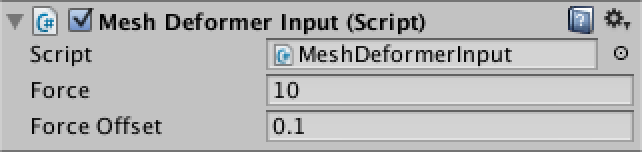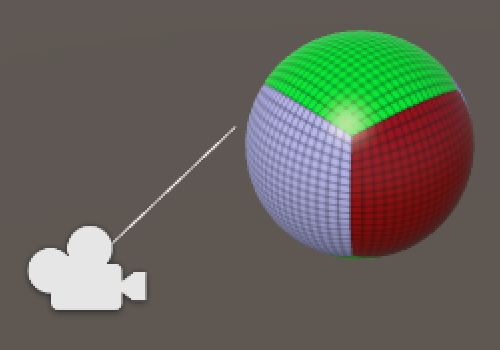# 第三部分基本变形

	public void AddDeformingForce (Vector3 point, float force) {
for (int i = 0; i < displacedVertices.Length; i++) {
}
}

void AddForceToVertex (int i, Vector3 point, float force) {
}

	void AddForceToVertex (int i, Vector3 point, float force) {
Vector3 pointToVertex = displacedVertices[i] - point;
}

Fv=Fd2Fv=Fd2.

Fv=F1+d2Fv=F1+d2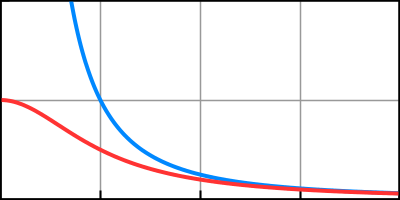Vector3 pointToVertex = displacedVertices[i] - point;
float attenuatedForce = force / (1f + pointToVertex.sqrMagnitude);

实际上，力首先被转换为加速度通道：

a=Fma=Fm.然后发现速度的变化：Δv=aΔtΔv=aΔt.为了保持简单，我们将忽略质量，就好像它是每个顶点一样

Δv=FΔtΔv=FΔt.

Vector3 pointToVertex = displacedVertices[i] - point;
float attenuatedForce = force / (1f + pointToVertex.sqrMagnitude);
float velocity = attenuatedForce * Time.deltaTime;

Vector3 pointToVertex = displacedVertices[i] - point;
float attenuatedForce = force / (1f + pointToVertex.sqrMagnitude);
float velocity = attenuatedForce * Time.deltaTime;
vertexVelocities[i] += pointToVertex.normalized * velocity;

	void Update () {
for (int i = 0; i < displacedVertices.Length; i++) {
UpdateVertex(i);
}
deformingMesh.vertices = displacedVertices;
deformingMesh.RecalculateNormals();
}

Δp=vΔtΔp=vΔt.

	void UpdateVertex (int i) {
Vector3 velocity = vertexVelocities[i];
displacedVertices[i] += velocity * Time.deltaTime;
}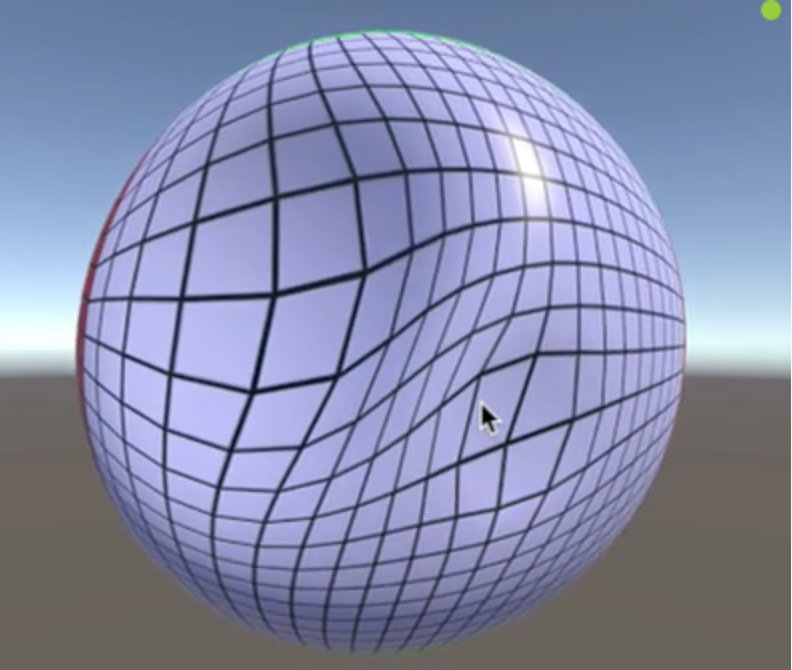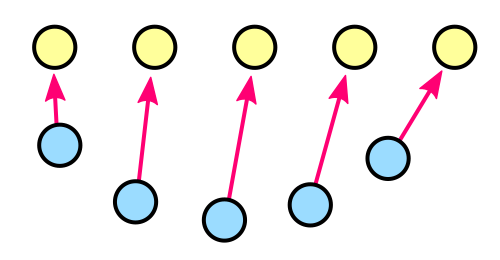public float springForce = 20f;

void UpdateVertex (int i) {
Vector3 velocity = vertexVelocities[i];
Vector3 displacement = displacedVertices[i] - originalVertices[i];
velocity -= displacement * springForce * Time.deltaTime;
vertexVelocities[i] = velocity;
displacedVertices[i] += velocity * Time.deltaTime;
}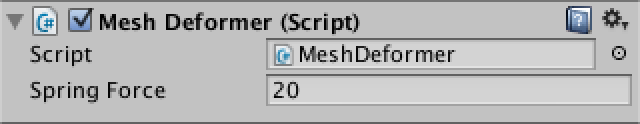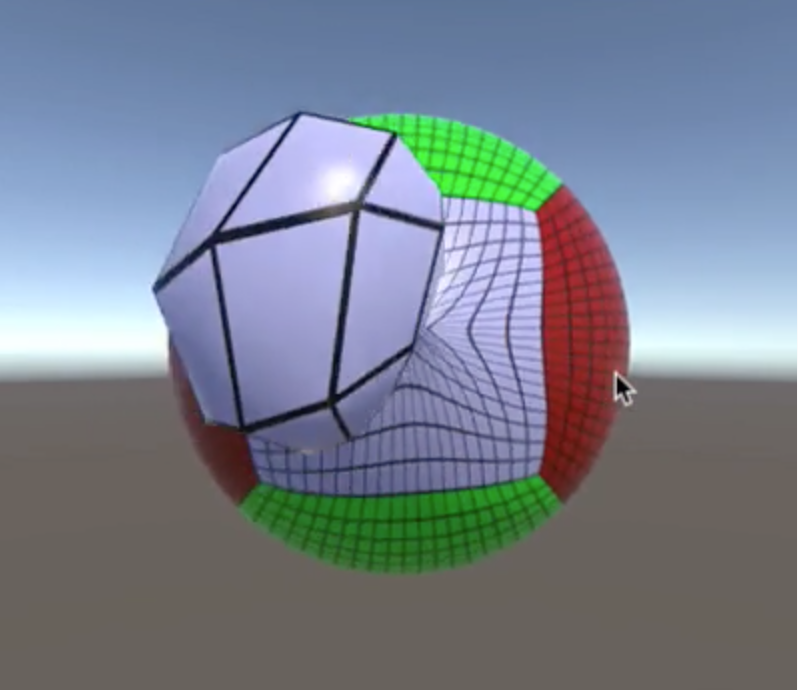vd=v(1dΔt)vd=v(1-dΔt).

	public float damping = 5f;

void UpdateVertex (int i) {
Vector3 velocity = vertexVelocities[i];
Vector3 displacement = displacedVertices[i] - originalVertices[i];
velocity -= displacement * springForce * Time.deltaTime;
velocity *= 1f - damping * Time.deltaTime;
vertexVelocities[i] = velocity;
displacedVertices[i] += velocity * Time.deltaTime;
}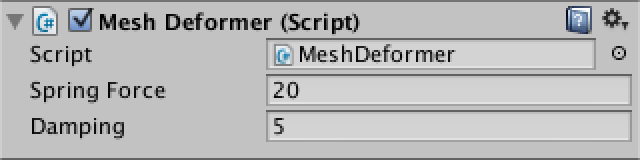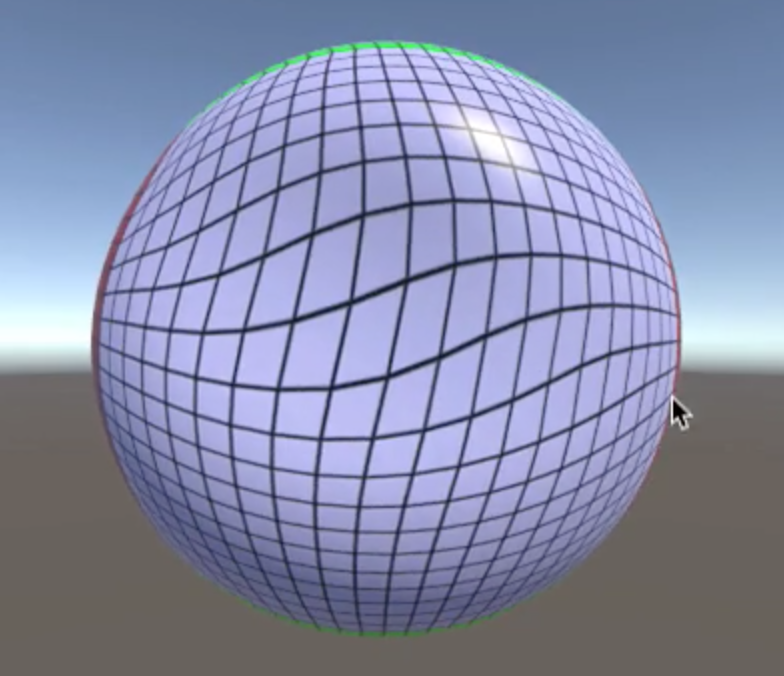public void AddDeformingForce (Vector3 point, float force) {
point = transform.InverseTransformPoint(point);
for (int i = 0; i < displacedVertices.Length; i++) {
}
}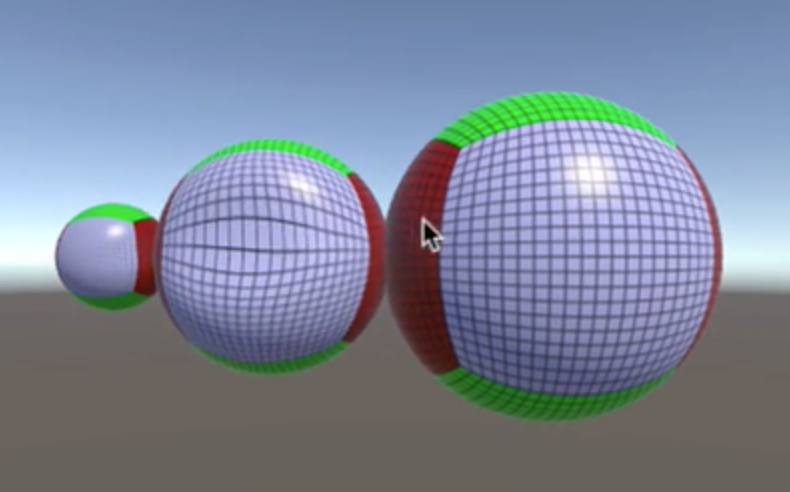float uniformScale = 1f;

void Update () {
uniformScale = transform.localScale.x;
…
}

	void AddForceToVertex (int i, Vector3 point, float force) {
Vector3 pointToVertex = displacedVertices[i] - point;
pointToVertex *= uniformScale;
float attenuatedForce = force / (1f + pointToVertex.sqrMagnitude);
float velocity = attenuatedForce * Time.deltaTime;
vertexVelocities[i] += pointToVertex.normalized * velocity;
}

void UpdateVertex (int i) {
Vector3 velocity = vertexVelocities[i];
Vector3 displacement = displacedVertices[i] - originalVertices[i];
displacement *= uniformScale;
velocity -= displacement * springForce * Time.deltaTime;
velocity *= 1f - damping * Time.deltaTime;
vertexVelocities[i] = velocity;
displacedVertices[i] += velocity * Time.deltaTime;
}

displacedVertices[i] += velocity * (Time.deltaTime / uniformScale);©️2019 CSDN 皮肤主题: 大白 设计师: CSDN官方博客